Homework Help Question & Answers

# Calculate the standard deviation for the two stocks

 Consider the following information:

 Probability of State Rate of Return if State Occurs Economy of Economy Stock A Stock B Recession .24 .055 –.34 Normal .64 .135 .24 Boom .12 .230 .47

 a. Calculate the expected return for the two stocks. (Do not round intermediate calculations and enter your answers as a percent rounded to 2 decimal places, e.g., 32.16.) b. Calculate the standard deviation for the two stocks. (Do not round intermediate calculations and enter your answers as a percent rounded to 2 decimal places, e.g., 32.16.)

#### Homework Answers

Answer #1 ✔ Recommended Answer

Consider the following information:

 Rate of Return if State Occurs State of Probability of State Economy of Economy Stock A Stock B Recession .24 .055 –.34 Normal .64 .135 .24 Boom .12 .230 .47

Requirement 1= Expected Return of=

Stock A= (0.24*0.055) + (0.64*0.135) + (0.12*0.230) = 12.72%

Stock B=(0.24*-0.34) + (0.64*0.24) + (0.12*0.47) = 12.84%

Requirement 2=The Standard Deviation for the two Stocks:

Stock A=

 Probability Rate of Return XP X2P P X 0.24 0.055 0.0132 0.000726 0.64 0.135 0.0864 0.011664 0.12 0.230 0.0276 0.006348 0.1272 0.018738

Standard Deviation = Square Root (0.018738- (0.1272)2 )

= Square Root (0.018738-0.01617984) = Square Root (0.00255816)

= 0.0505 = 5.05 %

Stock B=

 Probability Rate of Return XP X2P P X 0.24 -0.34 -0.0816 0.027744 0.64 0.24 0.1536 0.036864 0.12 0.47 0.0564 0.026508 0.1284 0.091116

Standard Deviation = Square Root (0.091116- (0.1284)2 )

= Square Root (0.091116-0.01648656) = Square Root (0.07462944)

= 0.27318 = 27.32 %

answered by: moon
Add a comment
Know the answer?
Your Answer:

#### Post as a guest

Your Name:

What's your source?

#### Earn Coin

Coins can be redeemed for fabulous gifts.

Similar Homework Help Questions
• ### A.) Calculate the expected return for the two stocks (Do not round intermediate calculations; enter your...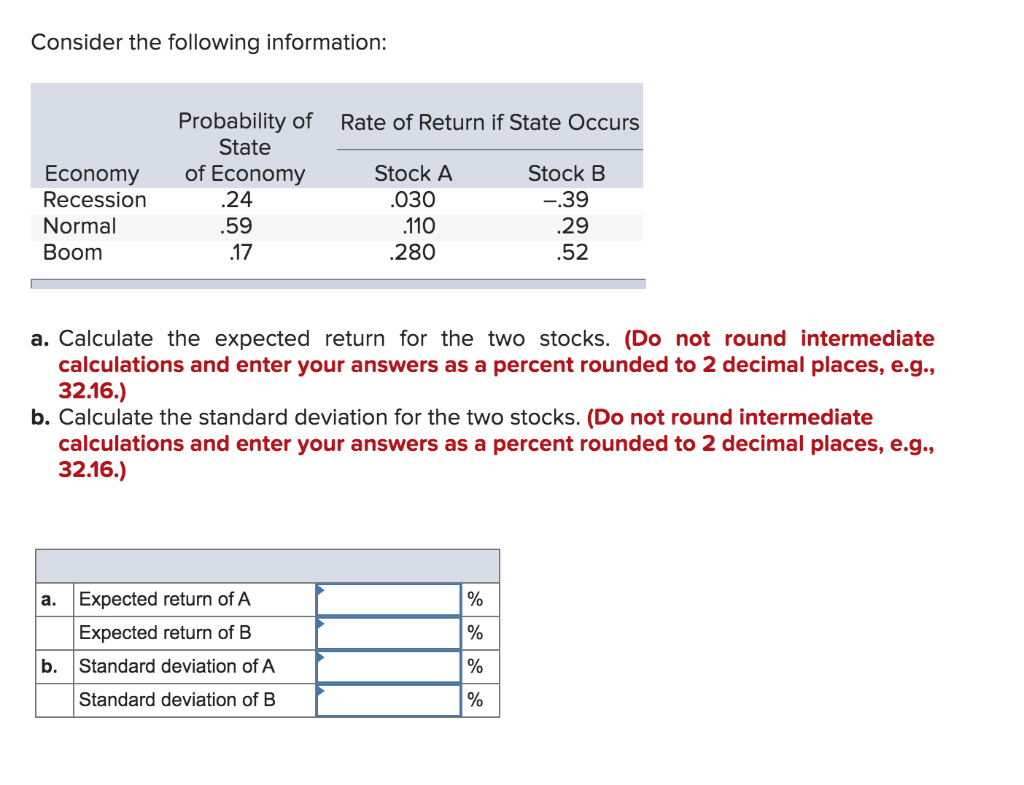A.) Calculate the expected return for the two stocks (Do not round intermediate calculations; enter your answers as a percent rounded to 2 decimal places). B.) Calculate the standard deviation for the two stocks (Do not round intermediate calculations; enter your answers as a percent rounded to 2 decimal places). Consider the following information: Probability of Rate of Return if State Occurs State of Economy Stock A Stock B .030 -.39 .59 110 .17 .280 .52 Economy Recession Normal Boom...

• ### Problem 13-7 Calculating Returns and Standard Deviations (LO1] Consider the following information: Rate of Return If...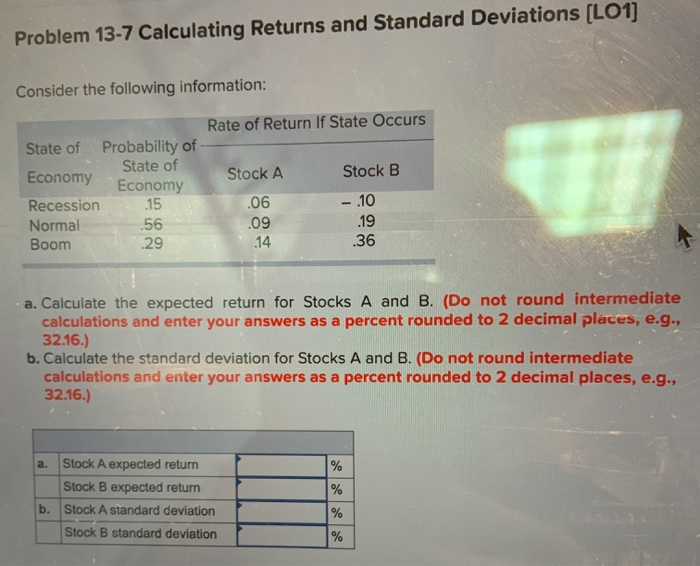Problem 13-7 Calculating Returns and Standard Deviations (LO1] Consider the following information: Rate of Return If State Occurs State of Probability of - State of Stock A Stock B Recession 15 - .10 Normal 56 .09 Boom Economy Economy .06 29 14 30 a. Calculate the expected return for Stocks A and B. (Do not round intermediate calculations and enter your answers as a percent rounded to 2 decimal places, e.g., 32.16.) b. Calculate the standard deviation for Stocks A...

• ### Consider the following information: Probability of Rate of Return if State Occurs State of Economy Economy...Consider the following information: Probability of Rate of Return if State Occurs State of Economy Economy Recession Stock A Stock B -35 25 20 010 Normal 55 090 Boom 25 240 48 a. Calculate the expected return for the two stocks. (Do not round intermediate calculations and enter your answers as a percent rounded to 2 decimal places, e.g. 32.16.) b. Calculate the standard deviation for the two stocks. (Do not round intermediate calculations and enter your answers as a...

• ### Consider the following information: Rate of Return If State Occurs State of Economy Probability of State...

Consider the following information: Rate of Return If State Occurs State of Economy Probability of State of Economy Stock A Stock B Recession .15 .06 −.10 Normal .56 .09 .19 Boom .29 .14 .36 Calculate the expected return for the two stocks. (Do not round intermediate calculations. Enter your answers as a percent rounded to 2 decimal places, e.g., 32.16.) Expected return Stock A % Stock B % Calculate the standard deviation for the two stocks. (Do not round intermediate...

• ### Consider the following information: Probability of Rate of Return if State Occurs State of Economy Stock...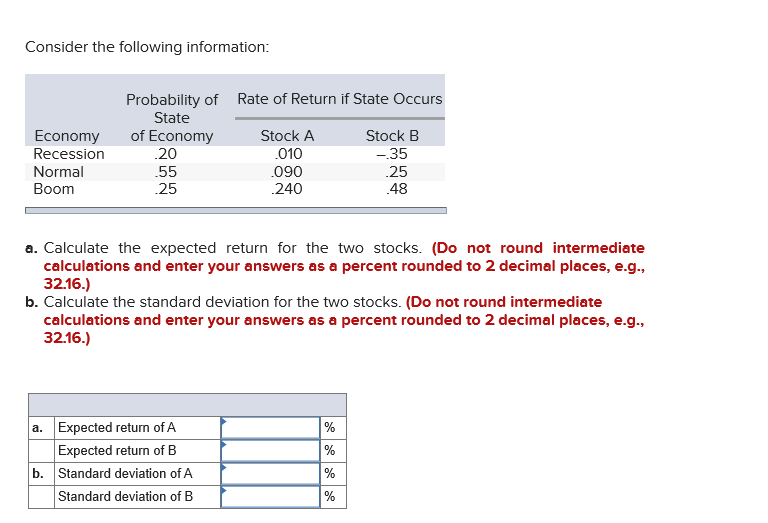Consider the following information: Probability of Rate of Return if State Occurs State of Economy Stock A Stock B .20 .010 090 .25 .240 48 Economy Recession Normal Boom -35 a. Calculate the expected return for the two stocks. (Do not round intermediate calculations and enter your answers as a percent rounded to 2 decimal places, e.g., 32.16.) b. Calculate the standard deviation for the two stocks. (Do not round intermediate calculations and enter your answers as a percent rounded...

• ### Consider the following information: Economy Rate of Return if State Occurs State of Probability of State...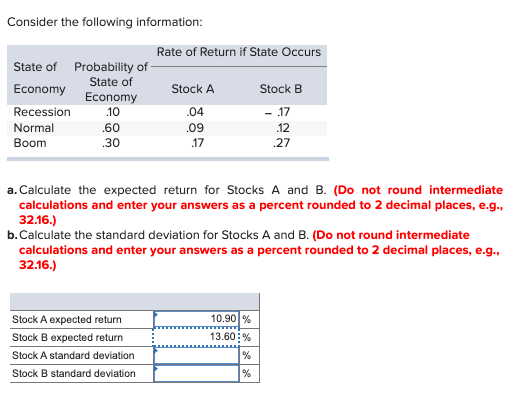Consider the following information: Economy Rate of Return if State Occurs State of Probability of State of Stock A Stock B Recession 10 .04 - 17 Normal .60 .09 Boom 30 27 Economy .12 .17 a. Calculate the expected return for Stocks A and B. (Do not round intermediate calculations and enter your answers as a percent rounded to 2 decimal places, e.g.. 32.16.) b. Calculate the standard deviation for Stocks A and B. (Do not round intermediate calculations and...

• ### Consider the following information: Rate of Return if State Occurs Probability of State of Economy Stock...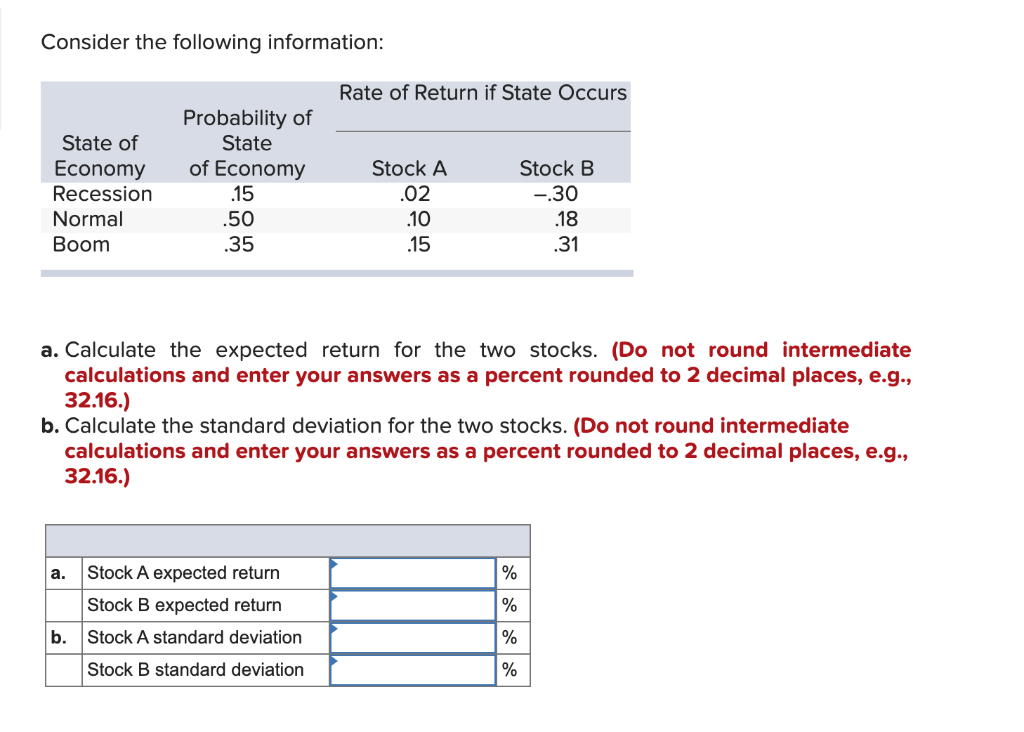Consider the following information: Rate of Return if State Occurs Probability of State of Economy Stock A Stock B State of Economy Recession Normal Boom .02 .15 .50 -30 .18 .35 .10 .15 .31 a. Calculate the expected return for the two stocks. (Do not round intermediate calculations and enter your answers as a percent rounded to 2 decimal places, e.g., 32.16.) b. Calculate the standard deviation for the two stocks. (Do not round intermediate calculations and enter your answers...

• ### Consider the following information: Economy Economy Rate of Return If State Occurs State of Probability of...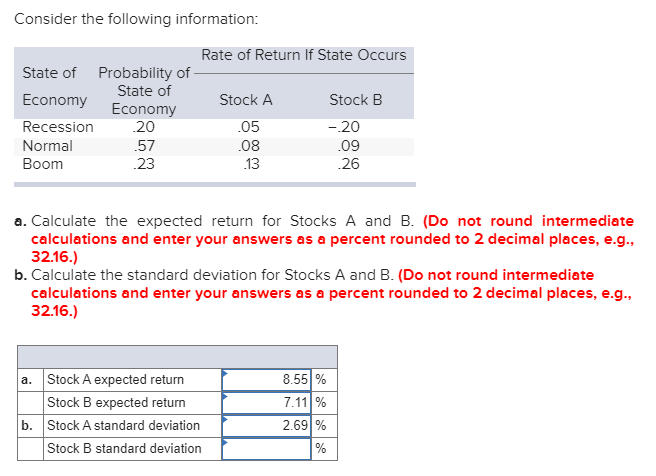Consider the following information: Economy Economy Rate of Return If State Occurs State of Probability of - State of Stock A Stock B Recession 20 .05 - 20 Normal 57 Boom 23 26 08 .09 a. Calculate the expected return for Stocks A and B. (Do not round intermediate calculations and enter your answers as a percent rounded to 2 decimal places, e.g., 32.16.) b. Calculate the standard deviation for Stocks A and B. (Do not round intermediate calculations and...

• ### Consider the following information: STOCK D Economy Recession Normal Boom Probability of State of Economy .23...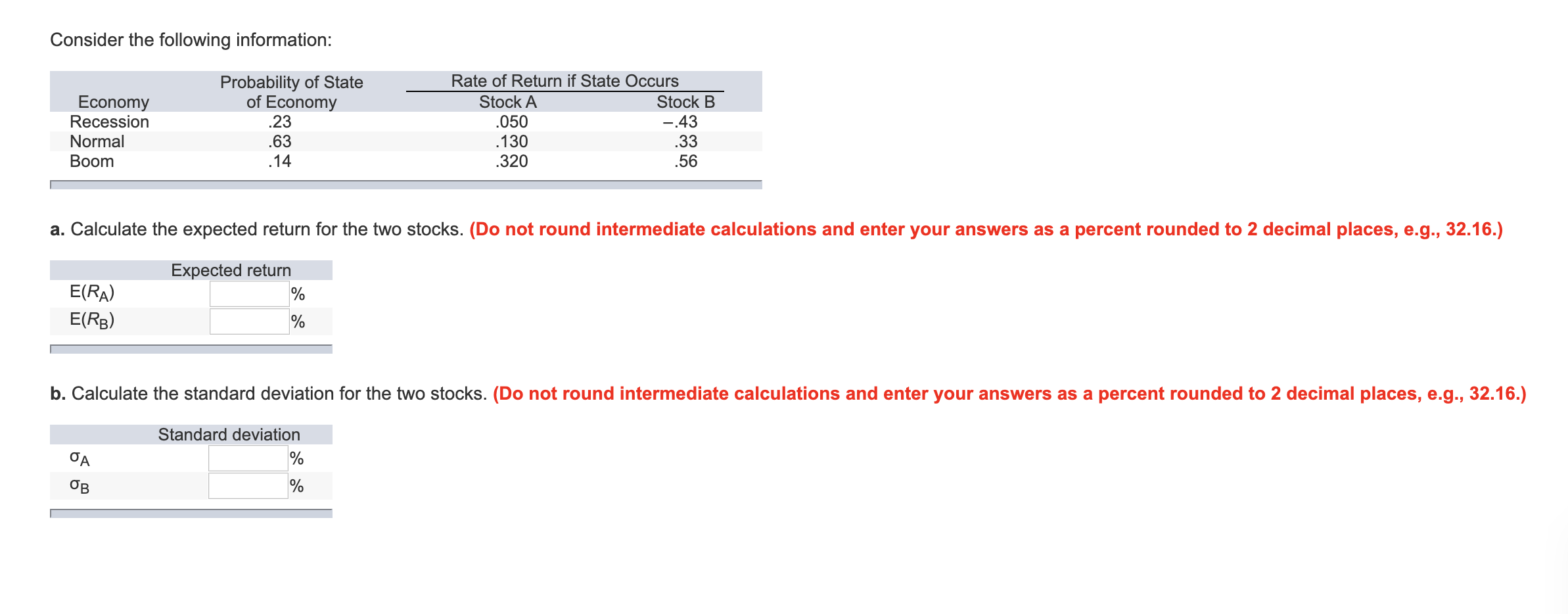Consider the following information: STOCK D Economy Recession Normal Boom Probability of State of Economy .23 .63 .14 Rate of Return if State Occurs Stock A Stock B .050 -.43 .130 .33 .320 .56 a. Calculate the expected return for the two stocks. (Do not round intermediate calculations and enter your answers as a percent rounded to 2 decimal places, e.g., 32.16.) Expected return E(RA) E(RB) b. Calculate the standard deviation for the two stocks. (Do not round intermediate calculations...

• ### Consider the following information: Probability of State of Economy Rate of Return of State Occurs Stock...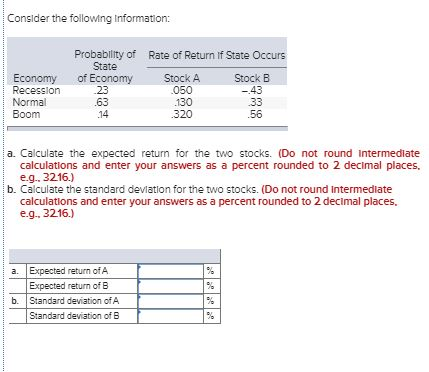Consider the following information: Probability of State of Economy Rate of Return of State Occurs Stock A Stock B 23 050 -43 Economy Recession Normal Boom 130 320 56 a. Calculate the expected return for the two stocks. (Do not round Intermediate calculations and enter your answers as a percent rounded to 2 decimal places, e.g., 32.16.) b. Calculate the standard deviation for the two stocks. (Do not round Intermediate calculations and enter your answers as a percent rounded to...

Free Homework App

Scan Your Homework
to Get Instant Free Answers
Need Online Homework Help?

Get Answers For Free
Most questions answered within 3 hours.